Next: Centralised moments Up: Non-orthogonal moments Previous: Non-orthogonal moments

## Cartesian moments

The discrete version of the Cartesian moment (Equation 1.13) for an image consisting of pixels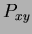, replacing the integrals with summations, is: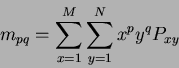(16)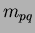Two dimensional Cartesian moment Where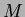and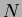are the image dimensions and the monomial product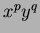is the basis function. Figure 1.2 illustrates the non-orthogonal (highly correlated) nature of these monomials (in contrast to the orthogonal polynomials in Figure 1.6, to be discussed later) plotted for the positiveaxis only.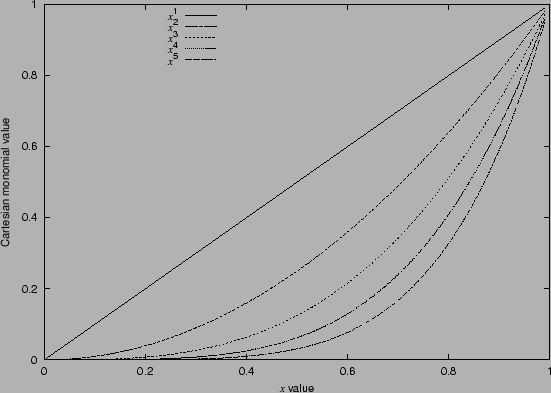The zero order moment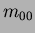is defined as the total mass (or power) of the image. If this is applied to a binary (i.e. a silhouette)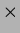image of an object, then this is literally a pixel count of the number of pixels comprising the object.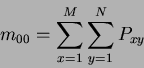(17)

The two first order moments are used to find the Centre Of Mass (COM)COMCentre of mass of an image. If this is applied to a binary image and the results are then normalised with respect to the total mass (), then the result is the centre co-ordinates of the object. Accordingly, the centre co-ordinates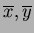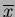axis centre of mass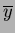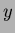axis centre of mass are given by :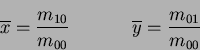(18)

The COM describes a unique position within the field of view which can then be used to compute the centralised moments of an image.Next: Centralised moments Up: Non-orthogonal moments Previous: Non-orthogonal moments
Jamie Shutler 2002-08-15